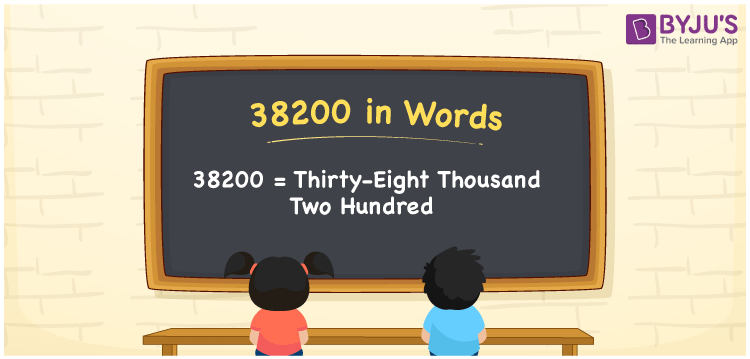# 38200 in Words

38200 in words is written as Thirty-eight thousand two hundred. In both the International System of Numerals and the Indian System of Numerals, 38200 is written as Thirty-eight thousand two hundred. The number 38200 is a Cardinal Number as it represents some quantity. For example, “that laptop costs 38200 rupees”.

 38200 in Words Thirty-eight thousand two hundred Thirty-eight thousand two hundred in Number 38200

## 38200 in English Words

38200 in English words is read as “Thirty-eight thousand two hundred.”## How to Write 38200 in Words?

To write 38200 in words, we shall use the place value chart. In the place value chart, put 3 in the ten thousands, 8 in the thousands, 2 in the hundreds and put 0 in the rest. Let us make a place value chart to write the number 38200 in words.

 Ten Thousands Thousands Hundreds Tens Ones 3 8 2 0 0

Thus, we can write the expanded form as

3 × Ten Thousand + 8 × Thousand + 2 × Hundred + 0 × Ten + 0 × One

= 3 × 10000 + 8 × 1000 + 2 × 100 + 0 × 10 + 0 × 1

= 30000 + 8000 + 200 + 0 + 0

= 38200

= Thirty-eight thousand two hundred.

38200 is a natural number, the successor of 38199 and the predecessor of 38201.

38200 in words – Thirty-eight thousand two hundred

• Is 38200 an odd number? – No
• Is 38200 an even number? – Yes
• Is 38200 a perfect square number? – No
• Is 38200 a perfect cube number? – No
• Is 38200 a prime number? – No
• Is 38200 a composite number? – Yes

## Frequently Asked Questions on 38200 in Words

Q1

### How to write 38200 in words?

38200 in words is written as Thirty-eight thousand two hundred.
Q2

### How to write 38200 in the International and Indian System of Numerals?

In both, the system of numerals, 38200 in words, is written as Thirty-eight thousand two hundred.
Q3

### How to write 38200 in a place value chart?

In the place value chart, write 3 in the ten thousands, 8 in the thousands, 2 in the hundreds and 0 in the tens and the ones, respectively.